# 四、检测和跟踪不同的身体部位

• 如何使用 Haar 级联
• 什么是完整的图像
• 什么是自适应增强
• 如何在实时视频流中检测和跟踪面部
• 如何在实时视频流中检测和跟踪眼睛
• 如何将太阳镜自动覆盖在人的脸上
• 如何检测眼睛，耳朵和嘴巴
• 如何使用形状分析检测瞳孔

# 什么是完整图片？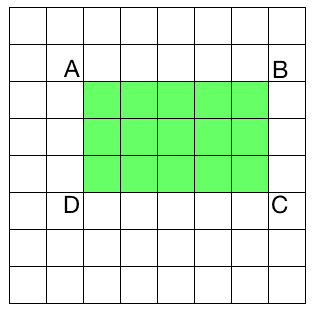矩形 ABCD 的面积 = AC - (AB + AD - AA)


# 检测和追踪人脸

OpenCV 提供了一个不错的人脸检测框架。 我们只需要加载级联文件并使用它来检测图像中的面部。 让我们看看如何做到这一点：

import cv2
import numpy as np

cap = cv2.VideoCapture(0)
scaling_factor = 0.5

while True:
frame = cv2.resize(frame, None, fx=scaling_factor,
fy=scaling_factor, interpolation=cv2.INTER_AREA)

for (x,y,w,h) in face_rects:
cv2.rectangle(frame, (x,y), (x+w,y+h), (0,255,0), 3)

cv2.imshow('Face Detector', frame)

c = cv2.waitKey(1)
if c == 27:
break

cap.release()
cv2.destroyAllWindows()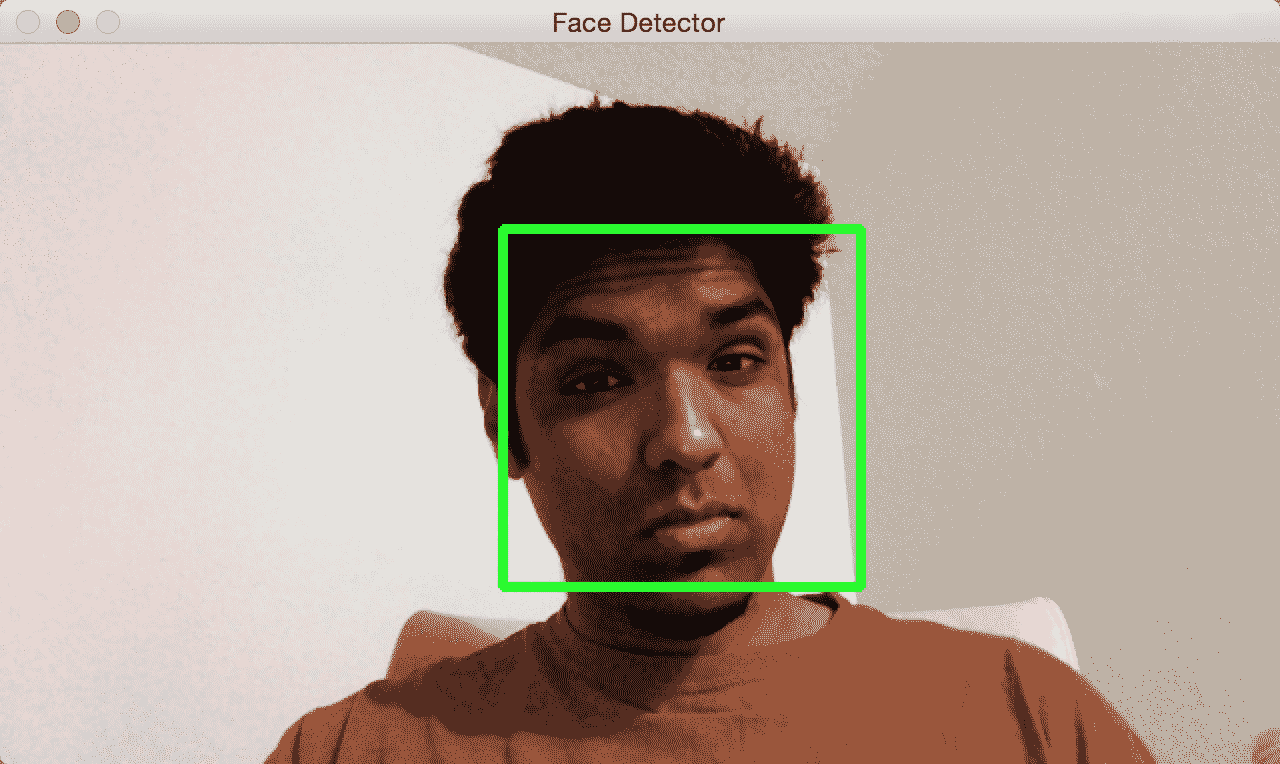# 人脸上的乐趣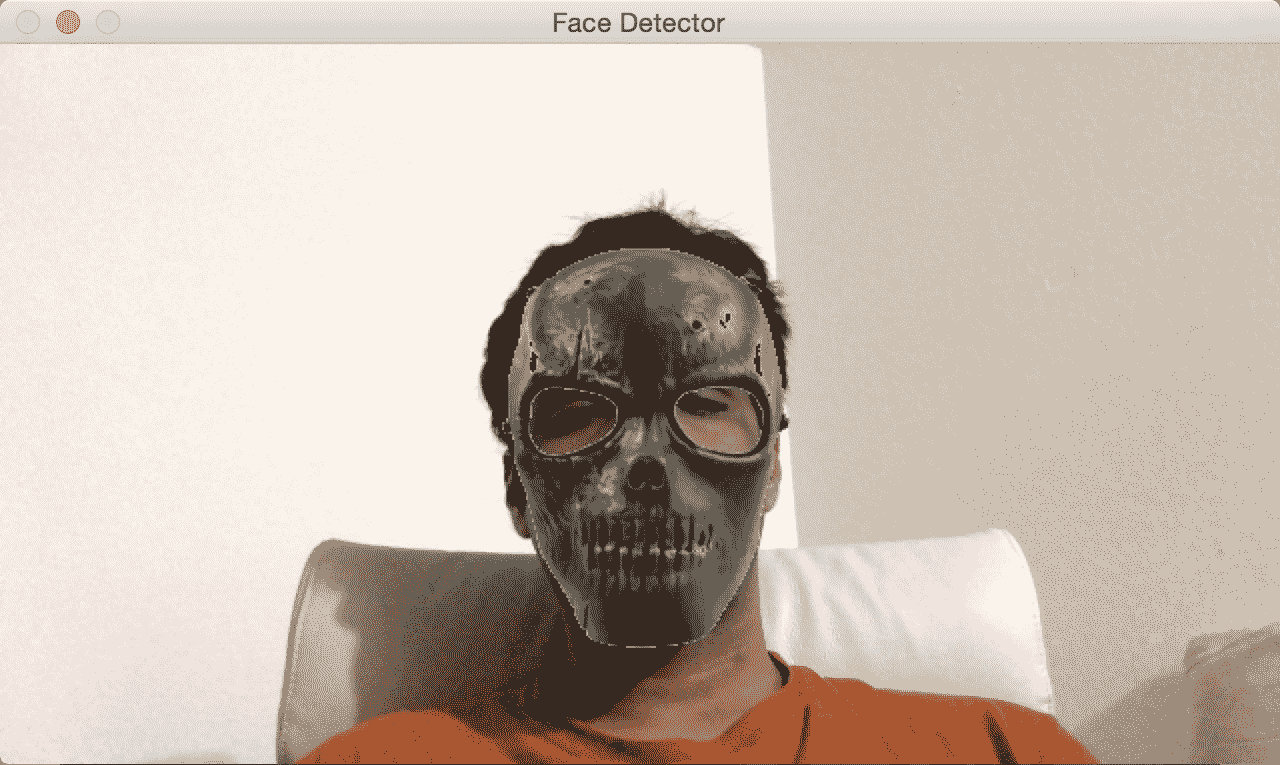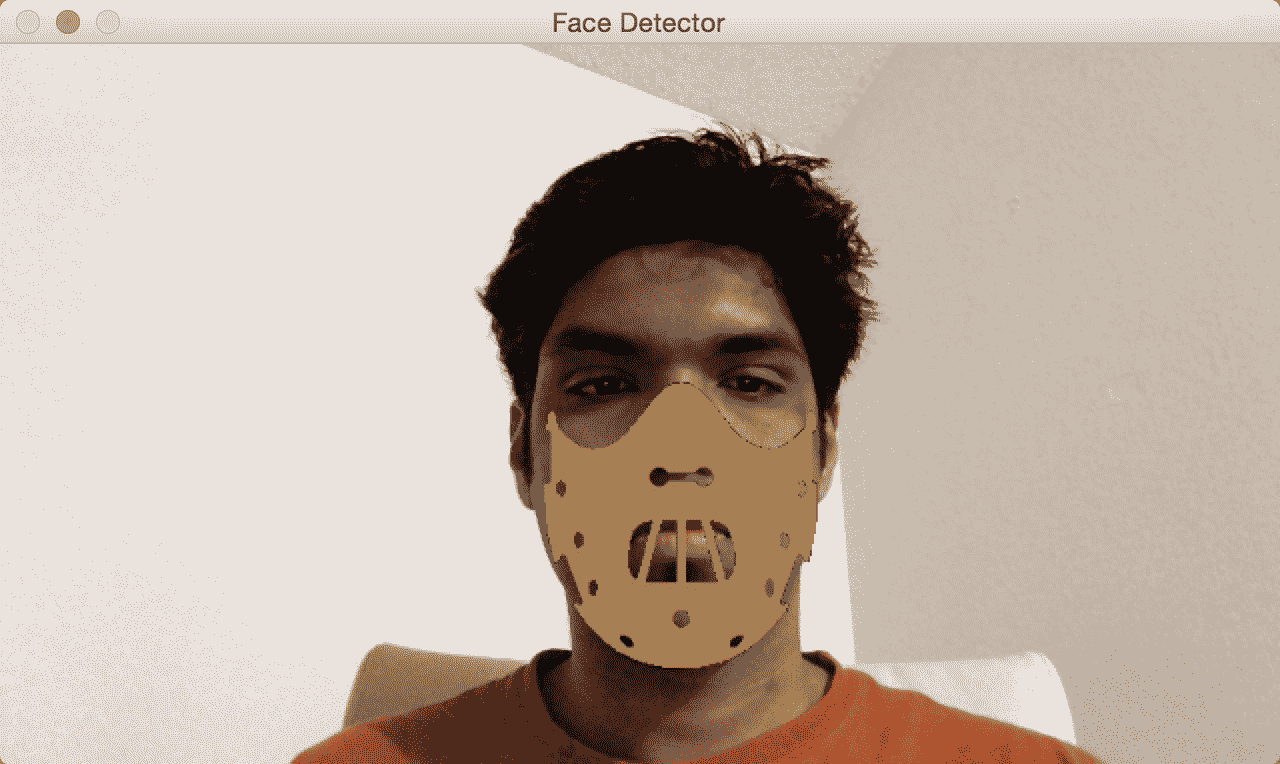import cv2
import numpy as np

cap = cv2.VideoCapture(0)
scaling_factor = 0.5

while True:
frame = cv2.resize(frame, None, fx=scaling_factor, fy=scaling_factor, interpolation=cv2.INTER_AREA)

for (x,y,w,h) in face_rects:
if h <= 0 or w <= 0: pass
# Adjust the height and weight parameters depending on the sizes and the locations.
# You need to play around with these to make sure you get it right.
h, w = int(1.0*h), int(1.0*w)
y -= int(-0.2*h)
x = int(x)

# Extract the region of interest from the image
frame_roi = frame[y:y+h, x:x+w]

# Convert color image to grayscale and threshold it

try:
# Use the mask to extract the face mask region of interest
# Use the inverse mask to get the remaining part of the image
except cv2.error as e:
print('Ignoring arithmentic exceptions: '+ str(e))

# add the two images to get the final output

cv2.imshow('Face Detector', frame)

c = cv2.waitKey(1)
if c == 27:
break

cap.release()
cv2.destroyAllWindows()


# 底层原理

frame_roi = frame[y:y+h, x:x+w]


# 从重叠图像中删除 Alpha 通道

import numpy as np
import cv2

def remove_alpha_channel(source, background_color):
source_img = cv2.cvtColor(source[:,:,:3], cv2.COLOR_BGR2GRAY)
source_mask = source[:,:,3] * (1 / 255.0)
bg_part = (255 * (1 / 255.0)) * (1.0 - source_mask)
weight = (source_img * (1 / 255.0)) * (source_mask)
dest = np.uint8(cv2.addWeighted(bg_part, 255.0, weight, 255.0, 0.0))
return dest

dest_img = remove_alpha_channel(orig_img)
cv2.imwrite('images/overlay_dest.png', dest_img, [cv2.IMWRITE_PNG_COMPRESSION])


# 检测眼睛

import cv2
import numpy as np

cap = cv2.VideoCapture(0)
ds_factor = 0.5

while True:
frame = cv2.resize(frame, None, fx=ds_factor, fy=ds_factor, interpolation=cv2.INTER_AREA)
gray = cv2.cvtColor(frame, cv2.COLOR_BGR2GRAY)

for (x,y,w,h) in faces:
roi_gray = gray[y:y+h, x:x+w]
roi_color = frame[y:y+h, x:x+w]
for (x_eye,y_eye,w_eye,h_eye) in eyes:
center = (int(x_eye + 0.5*w_eye), int(y_eye + 0.5*h_eye))
radius = int(0.3 * (w_eye + h_eye))
color = (0, 255, 0)
thickness = 3

cv2.imshow('Eye Detector', frame)

c = cv2.waitKey(1)
if c == 27:
break

cap.release()
cv2.destroyAllWindows()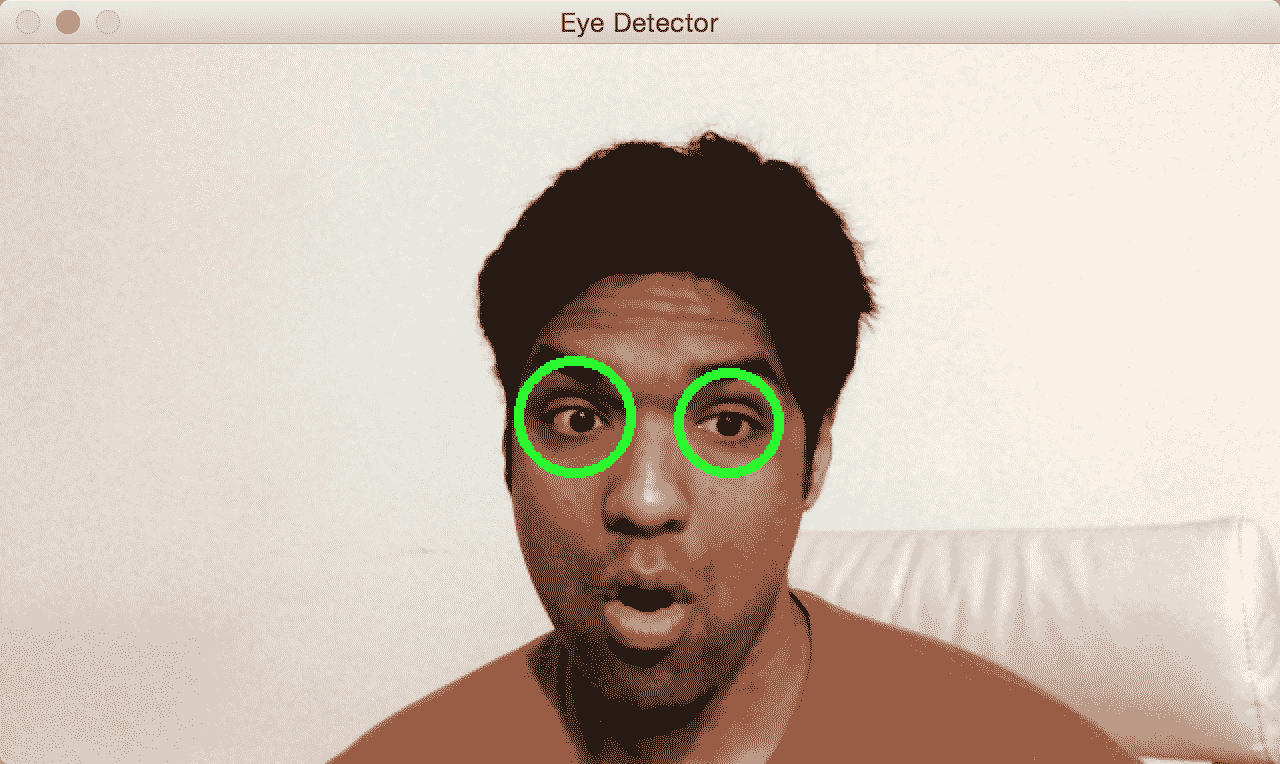# 有趣的眼睛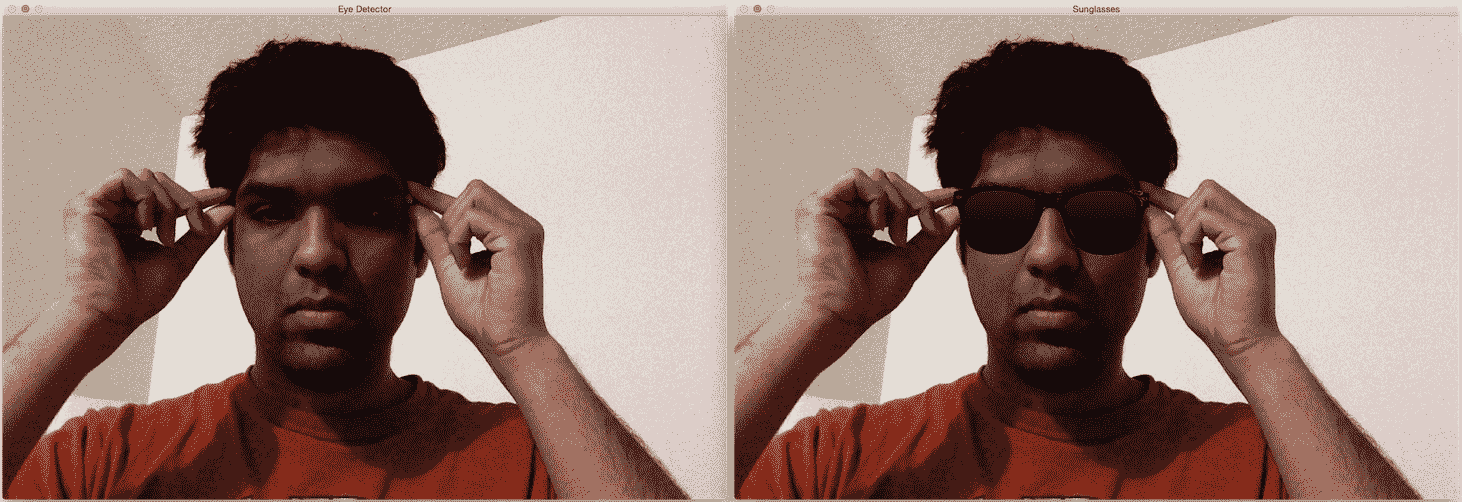import cv2
import numpy as np

cap = cv2.VideoCapture(0)

while True:
frame = cv2.resize(frame, None, fx=0.5, fy=0.5, interpolation=cv2.INTER_AREA)
vh, vw = frame.shape[:2]
vh, vw = int(vh), int(vw)

gray = cv2.cvtColor(frame, cv2.COLOR_BGR2GRAY)
centers = []

for (x,y,w,h) in faces:
roi_gray = gray[y:y+h, x:x+w]
roi_color = frame[y:y+h, x:x+w]
for (x_eye,y_eye,w_eye,h_eye) in eyes:
centers.append((x + int(x_eye + 0.5*w_eye), y + int(y_eye + 0.5*h_eye)))

if len(centers) > 1: # if detects both eyes
h, w = sunglasses_img.shape[:2]
# Extract the region of interest from the image
eye_distance = abs(centers - centers)
# Overlay sunglasses; the factor 2.12 is customizable depending on the size of the face
sunglasses_width = 2.12 * eye_distance
scaling_factor = sunglasses_width / w
print(scaling_factor, eye_distance)
overlay_sunglasses = cv2.resize(sunglasses_img, None, fx=scaling_factor, fy=scaling_factor, interpolation=cv2.INTER_AREA)

x = centers if centers < centers else centers

# customizable X and Y locations; depends on the size of the face
x -= int(0.26*overlay_sunglasses.shape)
y += int(0.26*overlay_sunglasses.shape)

h, w = overlay_sunglasses.shape[:2]
h, w = int(h), int(w)
frame_roi = frame[y:y+h, x:x+w]
# Convert color image to grayscale and threshold it
gray_overlay_sunglassess = cv2.cvtColor(overlay_sunglasses, cv2.COLOR_BGR2GRAY)
ret, mask = cv2.threshold(gray_overlay_sunglassess, 180, 255, cv2.THRESH_BINARY_INV)

try:
# Use the mask to extract the face mask region of interest
# Use the inverse mask to get the remaining part of the image
except cv2.error as e:
print('Ignoring arithmentic exceptions: '+ str(e))
#raise e

# add the two images to get the final output
else:
print('Eyes not detected')

cv2.imshow('Eye Detector', frame)
c = cv2.waitKey(1)
if c == 27:
break

cap.release()
cv2.destroyAllWindows()


# 检测耳朵

import cv2
import numpy as np

cap = cv2.VideoCapture(0)
scaling_factor = 0.5
while True:
frame = cv2.resize(frame, None, fx=scaling_factor, fy=scaling_factor, interpolation=cv2.INTER_AREA)
gray = cv2.cvtColor(frame, cv2.COLOR_BGR2GRAY)

for (x,y,w,h) in left_ear:
cv2.rectangle(frame, (x,y), (x+w,y+h), (0,255,0), 3)

for (x,y,w,h) in right_ear:
cv2.rectangle(frame, (x,y), (x+w,y+h), (255,0,0), 3)

cv2.imshow('Ear Detector', frame)
c = cv2.waitKey(1)
if c == 27:
break

cap.release()
cv2.destroyAllWindows()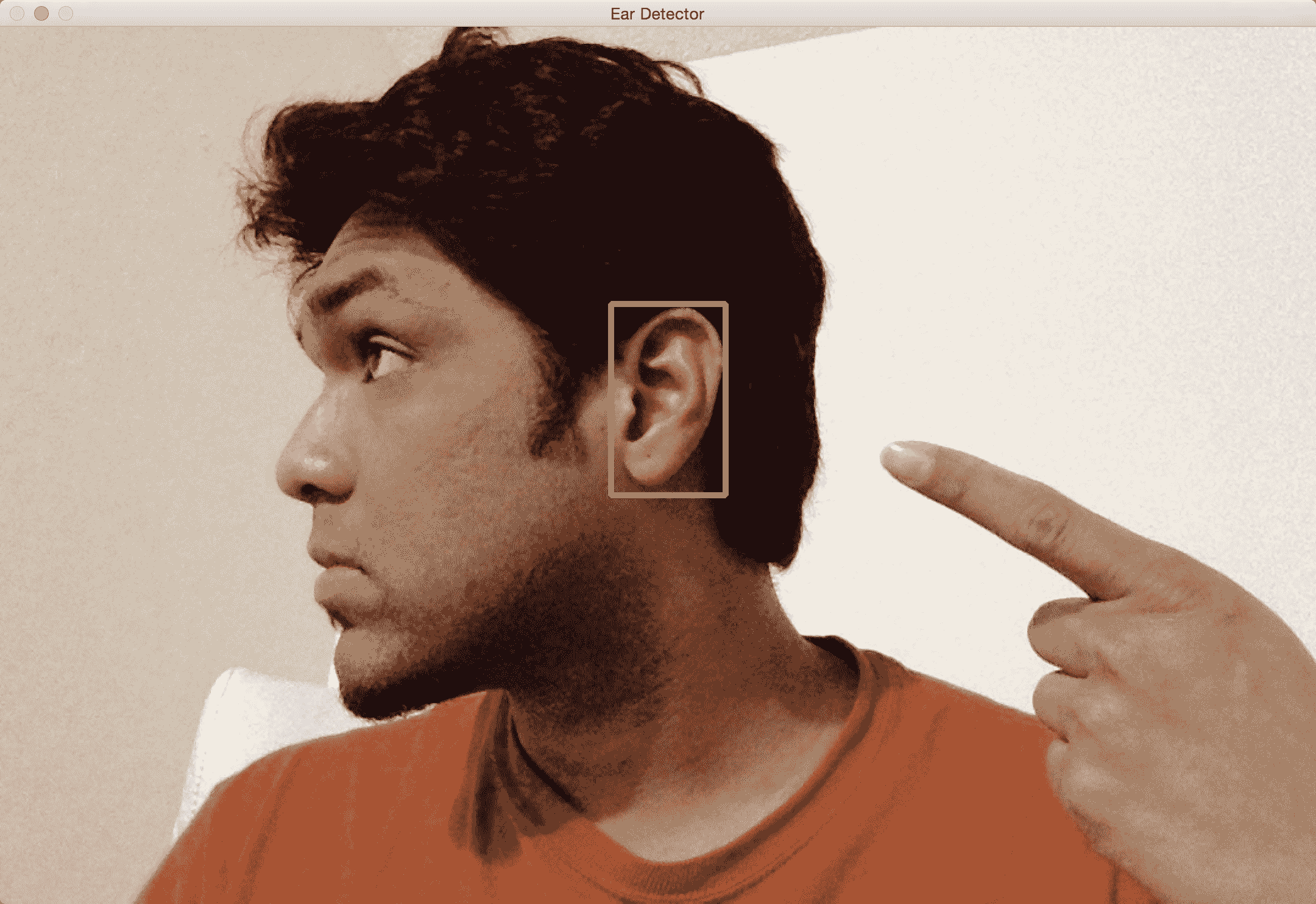# 检测嘴巴

import cv2
import numpy as np

cap = cv2.VideoCapture(0)
ds_factor = 0.5

while True:
frame = cv2.resize(frame, None, fx=ds_factor, fy=ds_factor, interpolation=cv2.INTER_AREA)
gray = cv2.cvtColor(frame, cv2.COLOR_BGR2GRAY)

for (x,y,w,h) in mouth_rects:
y = int(y - 0.15*h)
cv2.rectangle(frame, (x,y), (x+w,y+h), (0,255,0), 3)
break

cv2.imshow('Mouth Detector', frame)

c = cv2.waitKey(1)
if c == 27:
break

cap.release()
cv2.destroyAllWindows()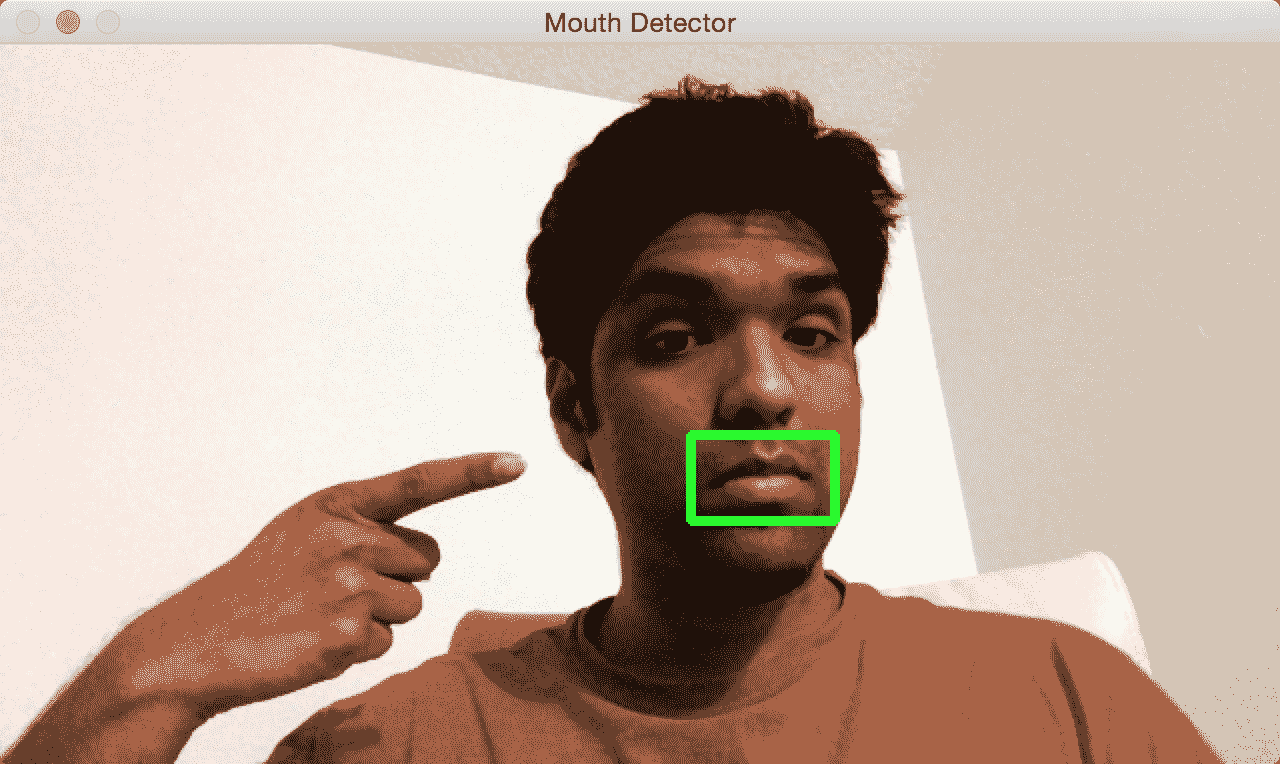# 覆盖胡须

import cv2
import numpy as np

cap = cv2.VideoCapture(0)
scaling_factor = 0.5

while True:
frame = cv2.resize(frame, None, fx=scaling_factor, fy=scaling_factor, interpolation=cv2.INTER_AREA)
gray = cv2.cvtColor(frame, cv2.COLOR_BGR2GRAY)

if len(mouth_rects) > 0:
(x,y,w,h) = mouth_rects
h, w = int(0.6*h), int(1.2*w)
x -= int(0.05*w)
y -= int(0.55*h)
frame_roi = frame[y:y+h, x:x+w]

cv2.imshow('Moustache', frame)
c = cv2.waitKey(1)
if c == 27:
break

cap.release()
cv2.destroyAllWindows()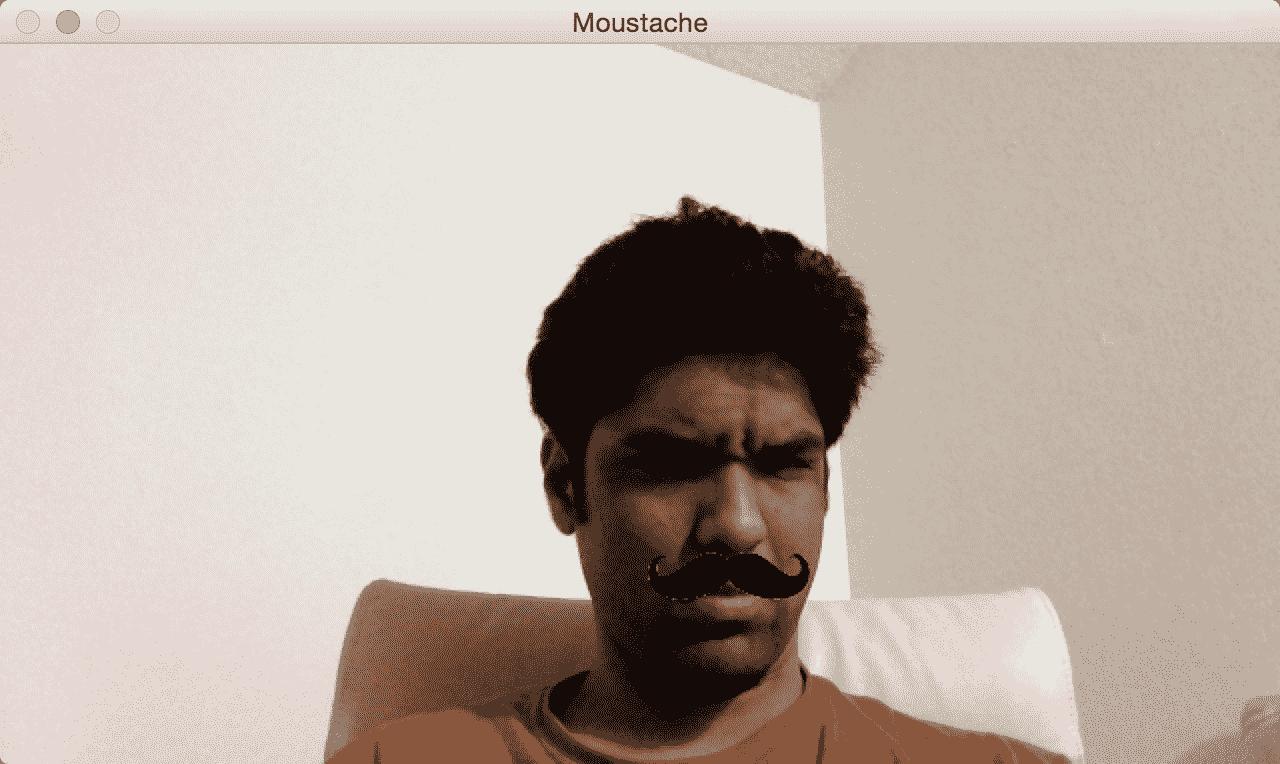# 检测瞳孔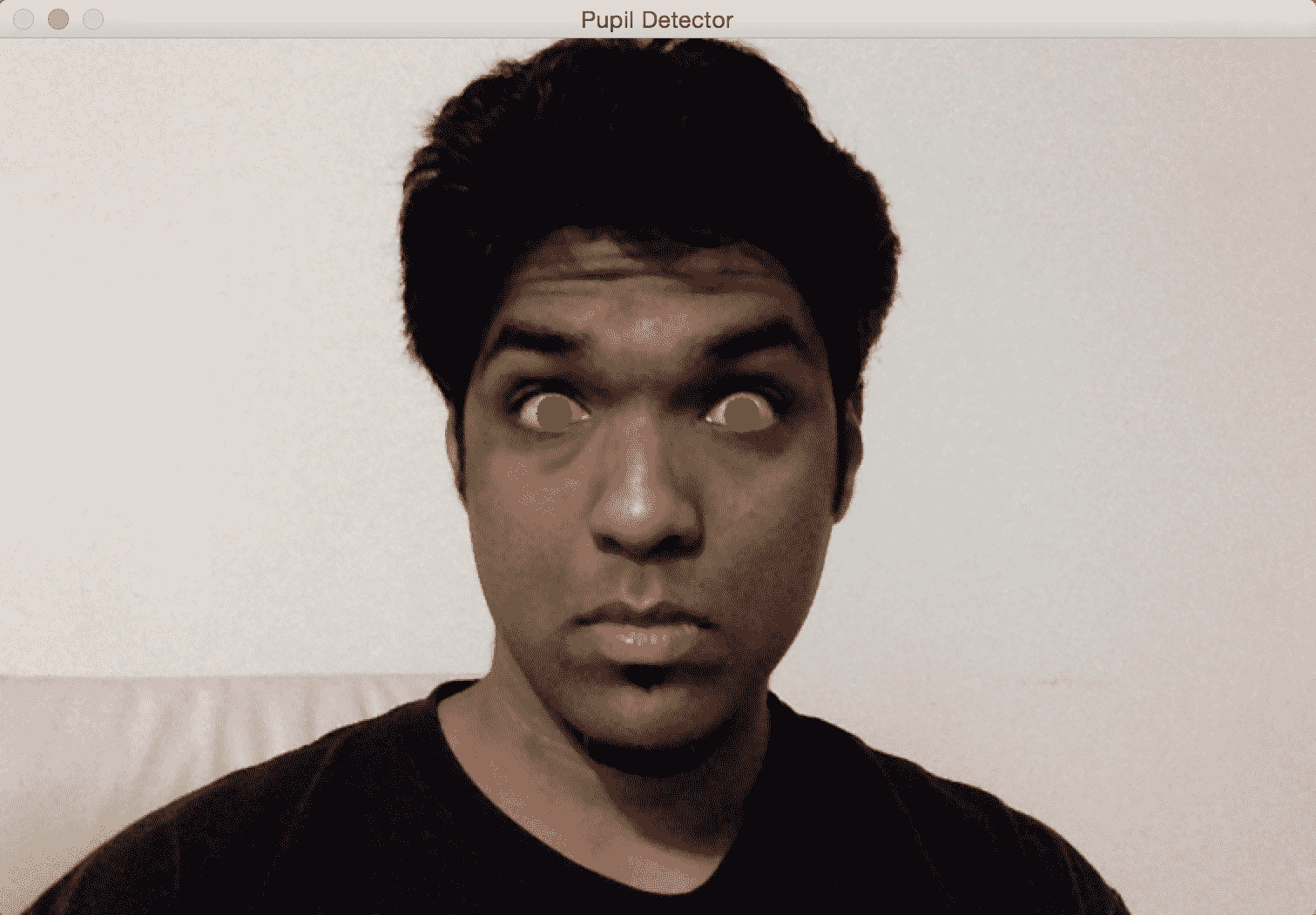import math

import cv2

cap = cv2.VideoCapture(0)
ds_factor = 0.5
contours = []

while True:
frame = cv2.resize(frame, None, fx=ds_factor, fy=ds_factor, interpolation=cv2.INTER_AREA)
gray = cv2.cvtColor(frame, cv2.COLOR_BGR2GRAY)
for (x_eye, y_eye, w_eye, h_eye) in eyes:
pupil_frame = gray[y_eye:y_eye + h_eye, x_eye:x_eye + w_eye]
ret, thresh = cv2.threshold(pupil_frame, 80, 255, cv2.THRESH_BINARY)
cv2.imshow("threshold", thresh)
im2, contours, hierarchy = cv2.findContours(thresh, cv2.RETR_LIST, cv2.CHAIN_APPROX_SIMPLE)
print(contours)

for contour in contours:
area = cv2.contourArea(contour)
rect = cv2.boundingRect(contour)
x, y, w, h = rect
radius = 0.15 * (w + h)

area_condition = (100 <= area <= 200)
symmetry_condition = (abs(1 - float(w)/float(h)) <= 0.2)
fill_condition = (abs(1 - (area / (math.pi * math.pow(radius, 2.0)))) <= 0.4)
cv2.circle(frame, (int(x_eye + x + radius), int(y_eye + y + radius)), int(1.3 * radius), (0, 180, 0), -1)

cv2.imshow('Pupil Detector', frame)
c = cv2.waitKey(1)
if c == 27:
break

cap.release()
cv2.destroyAllWindows()


# 解构代码

gray = cv2.cvtColor(img, cv2.COLOR_BGR2GRAY)